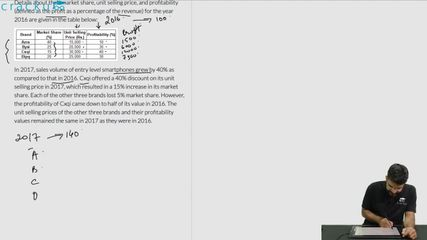### CAT 2018 Question Paper (Slot 2) Question 65

Instructions

There are only four brands of entry level smartphones called Azra, Bysi, Cxqi, and Dipq in a country.
Details about their market share, unit selling price, and profitability (defined as the profit as a percentage of the revenue) for the year 2016 are given in the table below:

In 2017, sales volume of entry level smartphones grew by 40% as compared to that in 2016. Cxqi offered a 40% discount on its unit selling price in 2017, which resulted in a 15% increase in its market share. Each of the other three brands lost 5% market share. However, the profitability of Cxqi came down to half of its value in 2016. The unit selling prices of the other three brands and their profitability values remained the same in 2017 as they were in 2016.

Question 65

# The brand that had the highest profit in 2017 is:

Solution

Let '100x' be the number of smartphones sold in year 2016. Then the number of smartphones sold in 2017 = 1.4*100x = 140x

It is given that Cxqi offered a 40% discount on its unit selling price in 2017 i.e. selling price in 2017 = 0.6*30000 = Rs. 18000

Also Cxqi's merket share increased by 15% whereas the other three brands lost 5% market share.

Amount of profit generated by Azra = $$\dfrac{10}{100}$$*15000*49x = 73500x

Amount of profit generated by Bysi = $$\dfrac{30}{100}$$*20000*28x = 168000x

Amount of profit generated by Cxqi = $$\dfrac{20}{100}$$*18000*42x = 151200x

Amount of profit generated by Dipq = $$\dfrac{30}{100}$$*25000*21x = 157500x

We can see that brand Bysi generated maximum profit in year 2017. Hence, option A is the correct answer.

### View Video Solution